Courses

# Pipelining - MCQ Quiz - 1

## 30 Questions MCQ Test RRB JE for Computer Science Engineering | Pipelining - MCQ Quiz - 1

Description
This mock test of Pipelining - MCQ Quiz - 1 for Computer Science Engineering (CSE) helps you for every Computer Science Engineering (CSE) entrance exam. This contains 30 Multiple Choice Questions for Computer Science Engineering (CSE) Pipelining - MCQ Quiz - 1 (mcq) to study with solutions a complete question bank. The solved questions answers in this Pipelining - MCQ Quiz - 1 quiz give you a good mix of easy questions and tough questions. Computer Science Engineering (CSE) students definitely take this Pipelining - MCQ Quiz - 1 exercise for a better result in the exam. You can find other Pipelining - MCQ Quiz - 1 extra questions, long questions & short questions for Computer Science Engineering (CSE) on EduRev as well by searching above.
QUESTION: 1

### The stage delays in a -stage pipeline are 800, 500, 400 and 300 picoseconds. The first stage (with delay 800 picoseconds) is replaced with a functionality equivalent design involving two stages with respective delays 600 and 350 picoseconds. The throughput increase of the pipeline is ___________ percent.

Solution:

In pipeline ideally CPI = 1
So in 1 cycle 1 instruction gets completed
Throughout is instructions in unit time
In pipeline 1, cycle time = max stage delay = 800psec
In 800 * psec, we expect to finish 1 instuction
So, in 1s, 1/800 instructions are expected to be completed, which is also the throughput for pipeline 1.
Similarly pipeline 2, throughput = 1/600
Throughput increase in percentage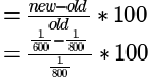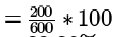= 33.33%

QUESTION: 2

### Consider a 3 GHz (gigahertz) processor with a three stage pipeline and stage latencies T1, T2 and T3 such that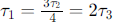If the longest pipeline stage is split into two pipeline stages of equal latency , the new frequency is __________ GHz,  ignoring delays in the pipeline registers.

Solution:

Given 3 stage pipeline , with 3 GHz processor.
Given , e 1 = 3 e2 / 4 = 2 e 3
Put e1 = 6x
we get, e2 = 8x , e 3 = 3x
Now largest stage time is 8x.
So, frequency is

1/8x

1/8x= 3 GHz
=>
1/x= 24 GHz ---------(1)

Now, we divide e 2 into two stages of 4x & 4x.
New processor has 4 stages -
6x , 4x, 4x, 3x.
Now largest stage time is 6x.
So, new frequency is
1\6x = 24/6= 4 GHz (Ans)
------- from (1)

QUESTION: 3

### The stage delays in a -stage pipeline are 800, 500, 400 and 300 picoseconds. The first stage (with delay 800 picoseconds) is replaced with a functionality equivalent design involving two stages with respective delays 600 and 350 picoseconds. The throughput increase of the pipeline is ___________ percent.

Solution:

In pipeline ideally CPI = 1
So in 1 cycle 1 instruction gets completed
Throughout is instructions in unit time
In pipeline 1, cycle time = max stage delay = 800psec
In 800 * psec, we expect to finish 1 instuction
So, in 1s, 1/800 instructions are expected to be completed, which is also the throughput for pipeline 1.
Similarly pipeline 2, throughput = 1/600
Throughput increase in percentage= 33.33%

QUESTION: 4

An instruction pipeline consists of 4 stages – Fetch (F), Decode field (D), Execute (E) and Result Write (W). The 5 instructions in a certain instruction sequence need these stages for the different number of clock cycles as shown by the table below
No. of cycles needed for
Instruction F  D  E  W

1                1  2  1  1
2                1  2  2  1
3                2  1  3  2
4                1  3  2  1
5                1  2  1  2
Find the number of clock cycles needed to perform the 5 instructions.

Solution:

answer = 15 cycles are required.QUESTION: 5

Comparing the time T1 taken for a single instruction on a pipelined CPU with time T2 taken on a non-pipelined but identicalCPU, we can say that

Solution:

Here we are comparing the execution time of only a single instruction. Pipelining in no way increases the execution time of a single instruction (the time from its start to end). It increases the overall performance by splitting the execution to multiple pipeline stages so that the following instructions can use the finished stages of the previous instructions. But in doing so pipelining causes some problems also as given in the below link, which might slow some instructions. So, (B) is the answer.

QUESTION: 6

An instruction pipeline has five stages where each stage take 2 nanoseconds and all instruction use all five stages. Branch instructions are not overlapped. i.e., the instruction after the branch is not fetched till the branch instruction is completed. Under ideal conditions,
a. Calculate the average instruction execution time assuming that 20% of all instructions executed are branch instruction. Ignore the fact that some branch instructions may be conditional.
b. If a branch instruction is a conditional branch instruction, the branch need not be taken. If the branch is not taken, the following instructions can be overlapped. When 80% of all branch instructions are conditional branch instructions, and 50% of the conditional branch instructions are such that the branch is taken, calculate the average instruction execution time.

Solution:

Each stage is 2ns. So, after 5 time units each of 2ns, the first instruction finishes (i.e., after 10ns), in every 2ns after that a new instruction gets finished. This is assuming no branch instructions. Now, once the pipeline is full, we can assume that the initial fill time doesn't matter our calculations and average execution time for each instruction is 2ns assuming no
branch instructions.
(a) Now, we are given that 20% of instructions are branch (like JMP) and when a branch instruction is executed, no further instruction enters the pipeline. So, we can assume every 5th instruction is a branch instruction. So, with this assumption, total time to finish 5 instruction will be 5 * 2 + 8 = 18 ns (as when a branch instruction enters the pipeline and before it finishes, 4 pipeline stages will be empty totaling 4 * 2 = 8 ns, as it is mentioned in question that the next instruction fetch starts only when branch instruction completes). And this is the same for every set of 5 instructions, and hence the average instruction execution time = 18/5 = 3.6 ns
(b) This is just a complex statement. But what we need is to identify the % of branch instructions which cause a branch to be taken as others will have no effect on the pipeline flow.
20% of branch instructions are branch instructions. 80% of branch instructions are conditional.
That means .2*.8 = 16% of instructions are conditional branch instructions and it is given that 50% of those result in a branch being taken.
So, 8% of instructions are conditional branches being taken and we also have 20% of 20% = 4% of unconditional branch
instructions which are always taken.
So, percentage of instructions where a branch is taken is 8+4 = 12% instead of 20% in (a) part.
So, in 100 instructions there will be 12 branch instructions. We can do a different calculation here as compared to (a) as 12 is not a divisor of 100. Each branch instruction causes a pipeline delay of 4*2 = 8 ns. So, 12 instructions will cause a
delay of 12 * 8 = 96 ns. For 100 instructions, we need 100 * 2 = 200 ns without any delay and with delay we require 200 + 96 = 296 ns for 100 instructions.
So, average instruction execution time = 296/100 = 2.96 ns (We can also use this method for part (a) which will give 100 * 2 + 20*8 = 360 ns for 100 instructions)

QUESTION: 7

Consider a 5-stage pipeline - IF (Instruction Fetch), ID (Instruction Decode and register read), EX (Execute), MEM (memory), and WB (Write Back). All (memory or register) reads take place in the second phase of a clock cycle and all writes occur in the first phase. Consider the execution of the following instruction sequence: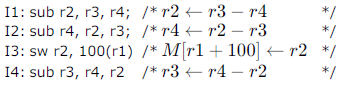a. Show all data dependencies between the four instructions.
b. Identify the data hazards.
c. Can all hazards be avoided by forwarding in this case.

Solution:

4 RAW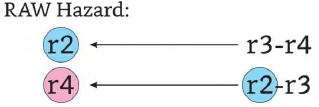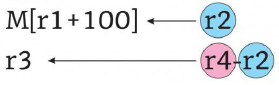3 WAR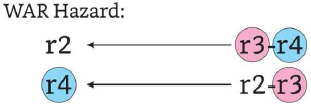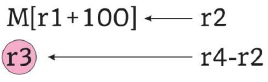With operand forwarding: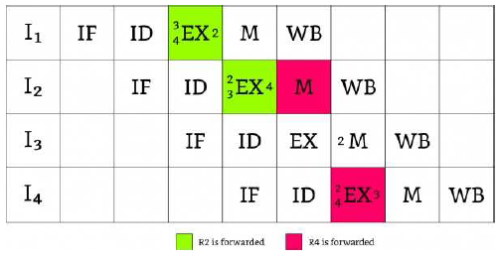Without it:
(both tables represent the same pipeline)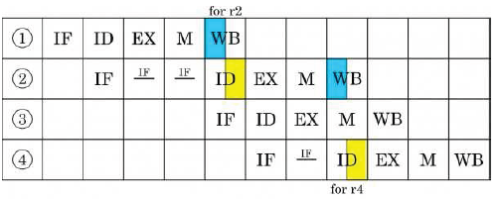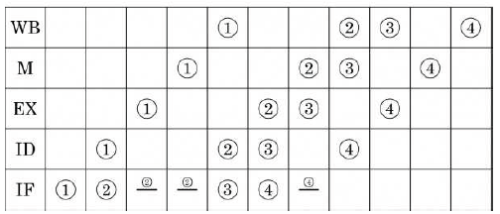QUESTION: 8

The performance of a pipelined processor suffers if

Solution:

A: Yes. Total delay = Max (All delays) + Register Delay.
B: Yes, if data forwarding is not there.
C: Yes, like ID and EX shares ID/EX register.

QUESTION: 9

For a pipelined CPU with a single ALU, consider the following situations
I. The j+1 –st instruction uses the result of the j-th instruction as an operand
II. The execution of a conditional jump instruction
III. The j-th and j+1st instructions require the ALU at the same time.

Which of the above can cause a hazard

Solution:

1. Data hazard
2. Control hazard
3. Structural hazard as only one ALU is there
So, D all of these.

QUESTION: 10

A 4-stage pipeline has the stage delays as 150, 120, 160 and 140 nanoseconds respectively. Registers that are usedbetween the stages have a delay of 5 nanoseconds each. Assuming constant clocking rate, the total time taken to process1000 data items on this pipeline will be

Solution:

Pipelining requires all stages to be synchronized meaning, we have to make the delay of all stages equal to the maximum pipeline stage delay which here is 160.
Time for execution of the first instruction = (160+5) * 3 + 160 = 655 ns (5 ns for intermediate registers which is not needed for the final stage).
Now, in every 165 ns, an instruction can be completed. So, Total time for 1000 instructions = 655 + 999*165 = 165.49 microseconds

QUESTION: 11

Consider a pipeline processor with 4 stages S1 to S4. We want to execute the following loop:
for (i = 1; i < = 1000; i++)
{I1, I2, I3, I4}
where the time taken (in ns) by instructions I1 to I4 for stages S1 to S4 are given below: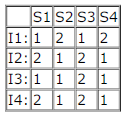The output of I1 for i = 2 will be available after

Solution: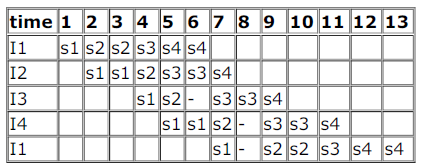so total time would be=13 ns
so option (c).

QUESTION: 12

A 5 stage pipelined CPU has the following sequence of stages:

• IF – instruction fetch from instruction memory
• RD – Instruction decode and register read
• EX – Execute: ALU operation for data and address computation
• MA – Data memory access – for write access, the register read at RD state is used.
• WB – Register write back

Consider the following sequence of instructions:

• I1: L R0, loc 1; R0 <= M[loc1]
• I2: A R0, R0; R0 <= R0 +R0
• I3: S R2, R0; R2 <= R2-R0

Let each stage take one clock cycle
What is the number of clock cycles taken to complete the above sequence of instructions starting from the fetch of ?

Solution:

8 cycles required with operand forwarding.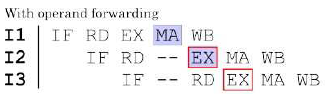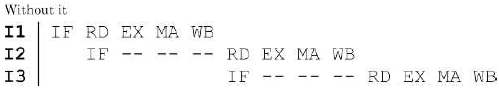it is not given that RD and WB stage could overlap.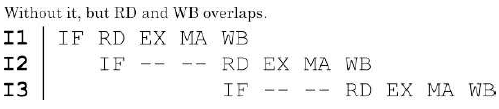QUESTION: 13

We have two designs D1 and D2 for a synchronous pipeline processor. D1 has 5 pipeline stages with execution times of 3nsec, 2 nsec, 4 nsec, 2 nsec and 3 nsec while the design D2 has 8 pipeline stages each with 2 nsec execution time Howmuch time can be saved using design D2 over design D1 for executing 100 instructions?

Solution:

(B) is the correct option for this question.
Execution time for Pipeline = (K+n-1)*execution_time where k = no of stages in pipeline n = no of instructions
execution time = Max(all stages execution time)
D1 = (5+100-1)*4 = 416
D2 = (8+100-1)*2 = 214
Time saved using D2 = 416-214 =202

QUESTION: 14

A CPU has a five-stage pipeline and runs at 1 GHz frequency. Instruction fetch happens in the first stage of the pipeline. A conditional branch instruction computes the target address and evaluates the condition in the third stage of the pipeline. The processor stops fetching new instructions following a conditional branch until the branch outcome is known. A program
executes 109 instructions out of which 20% are conditional branches. If each instruction takes one cycle to complete on average, the total execution time of the program is:

Solution:

Delay slots in pipeline caused due to a branch instruction is 2 as after the 3rd stage of current instruction (during 4th stage) IF of next begins. Ideally this should be during 2nd stage.
So, for total no. of instructions =109 and 20% branch, we have 0.2 x 2 x109 = 4 x 108 cycle penalty.
Since, clock speed is 1GHz and each instruction on average takes 1 cycle, total execution time in seconds will be

109/109+4x108/109 = 1.4

QUESTION: 15

A pipelined processor uses a 4-stage instruction pipeline with the following stages: Instruction fetch (IF), Instruction decode (ID), Execute (EX) and Writeback (WB). The arithmetic operations as well as the load and store operations are carried out in the EX stage. The sequence of instructions corresponding to the statement X = (S - R * (P + Q))/T is given below. The values of variables P, Q, R, S and T are available in the registers R0, R1, R2, R3 and R4 respectively, before the execution of the instruction sequence.
ADD R5, R0, R1 ; R5 ← R0 + R1
MUL R6, R2, R5 ; R6 ← R2 * R5
SUB R5, R3, R6 ; R5 ← R3 - R6
DIV R6, R5, R4 ; R6 ← R5/R4
STORE R6, X ; X ← R6
The number of Read-After-Write (RAW) dependencies, Write-After-Read( WAR) dependencies, and Write-After-Write (WAW) dependencies in the sequence of instructions are, respectively,

Solution:

(C) is the correct option for this question:
RAW
1. I1 - I2 (R5)
2. I2 - I3 (R6)
3. I3 - I4 (R5)
4. I4 - I5 (R6)
WAR
1. I2 - I3 (R5)
2. I3 - I4 (R6)
WAW
1. I1 - I3 (R5)
2. I2 - I4 (R6)

QUESTION: 16

A pipelined processor uses a 4-stage instruction pipeline with the following stages: Instruction fetch (IF), Instruction decode (ID), Execute (EX) and Writeback (WB). The arithmetic operations as well as the load and store operations are carried out in the EX stage. The sequence of instructions corresponding to the statement X = (S - R * (P + Q))/T is given below. The values of variables P, Q, R, S and T are available in the registers R0, R1, R2, R3 and R4 respectively, before the execution of the instruction sequence.
ADD R5, R0, R1 ; R5 → R0 + R1
MUL R6, R2, R5 ; R6 → R2 * R5
SUB R5, R3, R6 ; R5 → R3 - R6
DIV R6, R5, R4 ; R6 → R5/R4
STORE R6, X ; X ← R6
The IF, ID and WB stages take 1 clock cycle each. The EX stage takes 1 clock cycle each for the ADD, SUB and STORE operations, and 3 clock cycles each for MUL and DIV operations. Operand forwarding from the EX stage to the ID stage is used. The number of clock cycles required to complete the sequence of instructions is

Solution: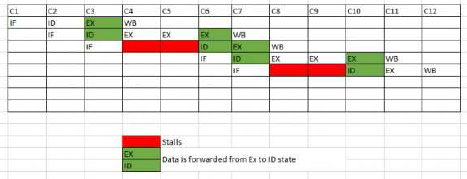This is what i have solved. so answer is 12

QUESTION: 17

Consider a pipelined processor with the following four stages:

• IF: Instruction Fetch
• ID: Instruction Decode and Operand Fetch
• EX: Execute
• WB: Write Back

The IF, ID and WB stages take one clock cycle each to complete the operation. The number of clock cycles for the EX stage depends on the instruction. The ADD and SUB instructions need 1 clock cycle and the MUL instruction needs 3 clock cycles in the EX stage. Operand forwarding is used in the pipelined processor. What is the number of clock cycles taken to complete the following sequence of instructions?
ADD   R2, R1, R0   R2 ← R1 + R0
MUL   R4, R3, R2   R4 ← R3 * R2
SUB   R6, R5, R4   R6 ← R5 - R4

Solution:

considering EX to EX data forwarding.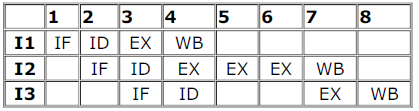QUESTION: 18

A processor takes 12 cycles to complete an instruction I. The corresponding pipelined processor uses 6 stages with the execution times of 3, 2, 5, 4, 6 and 2 cycles respectively. What is the asymptotic speedup assuming that a very large number of instructions are to be executed?

Solution:

For non pipeline processor we have n instruction and each instruction take 12 cycle so total 12n instruction.
For pipeline processor we have each stage strict to 6ns so time to complete the n instruction is 6*6+ (n-1)*6 Limn->∞ 12n / 36 + (n-1)*6 = 12 / 6 =2

QUESTION: 19

Which of the following are NOT true in a pipelined processor?
I. Bypassing can handle all RAW hazards
II. Register renaming can eliminate all register carried WAR hazards
III. Control hazard penalties can be eliminated by dynamic branch prediction

Solution:

(B) I and III
I - False Bypassing can't handle all RAW hazard, consider when any instruction depends on the result of LOAD
instruction, now LOAD updates register value at Memory Access Stage (MA), so data will not be available directly on
Execute stage.
II - True, register renaming can eliminate all WAR Hazard.
III- False, It cannot completely eliminate, though it can reduce Control Hazard Penalties

QUESTION: 20

Delayed branching can help in the handling of control hazardsFor all delayed conditional branch instructions, irrespective of whether the condition evaluates to true or false,

Solution:

76. Answer is A. In order to avoid the pipeline delay due to conditional branch instruction, a suitable instruction is placed
below the conditional branch instruction such that the instruction will be executed irrespective of whether branch is taken
or not and won't affect the program behaviour.
77. Answer is D) I4. The STORE instruction can be moved below the conditional branch instruction. Whether the branch is
taken or not, STORE will be executed as the next instruction after conditional branch instruction, due to delayed branching.
Here, I3 is not the answer because the branch conditional variable R1 is dependent on it. Same for I1. Similarly, I4 has a dependency on I2 and hence I2 must be executed before I4.

QUESTION: 21

Delayed branching can help in the handling of control hazards
The following code is to run on a pipelined processor with one branch delay slot:
I1: ADD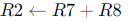I2: Sub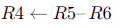I3: ADD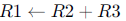I4: STORE Memory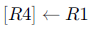BRANCH to Label if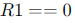Which of the instructions I1, I2, I3 or I4 can legitimately occupy the delay slot without any program modification?

Solution:

What is Delayed Branching ?
One way to maximize the use of the pipeline, is to find an instruction that can be safely exeucted whether the branch is taken or not, and execute that instruction. So, when a branch instruction is encountered, the hardware puts the instruction
following the branch into the pipe and begins executing it, just as in predict-not-taken. However, unlike in predict-nottaken, we do not need to worry about whether the branch is taken or not, we do not need to clear the pipe because no matter whether the branch is taken or not, we know the instruction is safe to execute.

Moving I1 after branch

• I1 is updating the value of R2
• R2 which is used to determine branch condition R1
• Value of R2 is available after branch

⇒ Cannot be moved

Moving I3 after branch

• value of R1 is computed in this instruction
• R1 is the branch condition

⇒ Cannot be moved
Moving I4 after branch

• I4 is simple store instruction used to store R1 in memory
• program execution will have no effect if this is placed after conditional branch

⇒ Cannot be moved
Moving I2 after branch

• It update the memory location to place the storing of conditional branch instruction R1
• If moved after branch , when compiler reaches I4 program execution will stop

⇒ Cannot be moved

• However I2 I4 both can be moved after the branch instruction

Apt choice will be I4

Hence Option D

QUESTION: 22

A non pipelined single cycle processor operating at 100 MHz is converted into a synchronous pipelined processor with five stages requiring 2.5 nsec, 1.5 nsec, 2 nsec, 1.5 nsec and 2.5 nsec, respectively. The delay of the latches is 0.5 nsec. The speedup of the pipeline processor for a large number of instructions is

Solution:

explanation:
for non pipeline system time required = 2.5 + 1.5 + 2.0 + 1.5 + 2.5 = 10
for pipelined system = max(stage delay) + max(latch delay) = 2.5 + 0.5 = 3 speedup = time in non pipelie / time in pipeline = 10/3 = 3.33

QUESTION: 23

Consider a 4 stage pipeline processor. The number of cycles needed by the four instructions I1, I2, I3, I4 in stages S1, S2,
S3, S4 is shown below: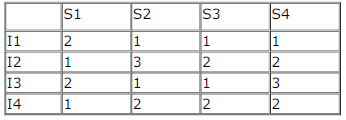What is the number of cycles needed to execute the following loop?
For (i=1 to 2) {I1; I2; I3; I4;}

Solution:

Here bound of the loop are constants, therefore compiler will do the loop unrolling(If compiler won't then prefetcher will do) to increase the instruction level parallelism. And after loop unrolling 23 cycles are required for execution. Therefore correct answer would be (B).
PS: We assume the buffers between the pipeline stages can store multiple results in the form of a queue.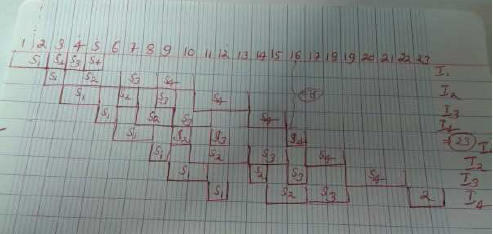QUESTION: 24

A 5-stage pipelined processor has Instruction Fetch (IF), Instruction Decode (ID), Opearnd Fetch (OF), Perform Operation (PO) and Write Operand (WO) stages. The IF, ID, OF and WO stages take 1 clock cycle each for any instruction. The PO stage takes 1 clock cycle for ADD and SUB instructions, 3 clock cycles for MUL instruction and 6 clock cycles for DIV instruction respectively. Operand forwarding is used in the pipeline. What is the number of clock cycles needed to execute the following sequence of instructions?

Instruction             Meaning of instruction

t0 : MUL R2,R0,R1R2 ← R0 ∗ R1
t1 : DIV R5,R3,R4 R5 ← R3/R4
t2 : ADD R2,R5,R2R2 ← R5 + R2
t3 : SUB R5,R2,R6 R ← − 5 R2 R6

Solution: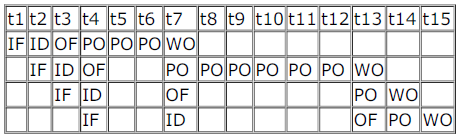Operand forwarding allows an output to be passed for the next instruction. Here from the output of PO stage of DIV instruction operand is forwarded to the PO stage of ADD instruction and similarly between ADD and SUB instructions.
Hence, 15 cycles required.

QUESTION: 25

Consider an instruction pipeline with four stages (S1, S2, S3 and S4) each with combinational circuit only. The pipeline registers are required between each stage and at the end of the last
stage. Delays for the stages and for the pipeline registers are as given in the figure.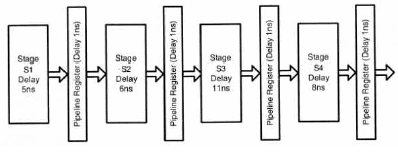What is the approximate speed up of the pipeline in steady state under ideal conditions when compared to the corresponding non-pipeline implementation?

Solution:

In pipeline system Time taken is determined by the max delay at any stage i.e., 11ns plus the delay incurred by pipeline
stages i.e., 1ns = 12ns. In non-pipeline system Delay = 5ns + 6ns + 11ns + 8ns = 30ns.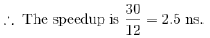QUESTION: 26

Register renaming is done in pipelined processors

Solution:

Register renaming is done to eliminate WAR (Write after Read) and WAW (Write after Write) dependency between instructions which could have caused pipieline stalls. Hence (C) is the answer.
Example:
I2: Write C to A
Here, there is a WAR dependency and pipeline would need stalls. In order to avoid it register renaming is done and Write C to A will be
Write C to A' WAR dependency is actually called anti-dependency and there is no real dependency except the fact that both uses same memory location. Register renaming can avoid this. Similarly WAW also.

QUESTION: 27

Consider an instruction pipeline with five stages without any branch prediction: Fetch Instruction (FI), Decode Instruction(DI), Fetch Operand (FO), Execute Instruction (EI) and Write Operand (WO). The stage delays for FI, DI, FO, EI and WO are5 ns, 7 ns, 10 ns, 8 ns and 6 ns, respectively. There are intermediate storage buffers after each stage and the delay of eachbuffer is 1 ns. A program consisting of 12 instructions I1, I2, I3, …, I12 is executed in this pipelined processor. Instruction I4is the only branch instruction and its branch target is I9. If the branch is taken during the execution of this program, thetime (in ns) needed to complete the program is

Solution:

After pipelining we have to adjust the stage delays such that no stage will be waiting for another to ensure smooth pipelining (continuous flow). Since we can not easily decrease the stage delay, we can increase all the stage delays to the maximum delay possible. So, here maximum delay is 10ns. Buffer delay given is 1ns. So, each stage takes 11ns in total.
FI of I9 can start only after the EI of I4. So, the total execution time will be

15 x 11 = 165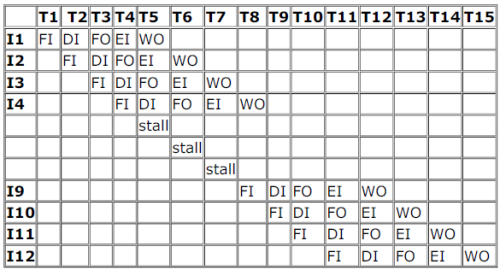QUESTION: 28

Consider a 6-stage instruction pipeline, where all stages are perfectly balanced. Assume that there is no cycle-time overhead of pipelining. When an application is executing on this 6-stage pipeline, the speedup achieved with respect to non-pipelined execution if 25% of the instructions incur 2 pipeline stall cycles is ____________

Solution:

Time without pipeline = 6 stages=6 cycles
Time with pipeline =1+stall freqency*stall cycle
=1+.25*2
=1.5

Speed up = 6/1.5
=4

QUESTION: 29

An instruction pipeline has five stages, namely, instruction fetch (IF), instruction decode and register fetch (ID/RF), instruction execution (EX), memory access (MEM), and register writeback (WB) with stage latencies 1 ns, 2.2 ns, 2 ns, 1 ns, and 0.75 ns, respectively (ns stands for nanoseconds). To gain in terms of frequency, the designers have decided to split the ID/RF stage into three stages (ID, RF1, RF2) each of latency 2.2/3 ns. Also, the EX stage is split into two stages (EX1, EX2) each of latency 1 ns. The new design has a total of eight pipeline stages. A program has 20% branch instructions which execute in the EX stage and produce the next instruction pointer at the end of the EX stage in the old design and at the end of the EX2 stage in the new design. The IF stage stalls after fetching a branch instruction until the next instruction pointer is computed. All instructions other than the branch instruction have an average CPI of one in both the designs. The execution times of this program on the old and the new design are P and Q nanoseconds, respectively. The value of P/Q is __________.

Solution:

cpi for first case = 2.2(1+2*.2) as the stall required is 2 and 2.2 is the maximum stage delay.
cpi for second state = 1*(1+5*.2) as now stall increase to 5 as there are five stages before the address is calculated and
the maximum stage delay now is 1.
cpu_time1/cpu_time2 = 3.08/2 = 1.54

QUESTION: 30

Consider the following processors (ns stands for nanoseconds). Assume that the pipeline registers have zero latency.
P1: Four-stage pipeline with stage latencies 1 ns, 2 ns, 2 ns, 1 ns.
P2: Four-stage pipeline with stage latencies 1 ns, 1.5 ns, 1.5 ns, 1.5 ns.
P3: Five-stage pipeline with stage latencies 0.5 ns, 1 ns, 1 ns, 0.6 ns, 1 ns.
P4: Five-stage pipeline with stage latencies 0.5 ns, 0.5 ns, 1 ns, 1 ns, 1.1 ns.
Which processor has the highest peak clock frequency?

Solution:

frequency = 1 / max( time in stages)
for P3 it is 1/1 GHz
for P1 it is 1/2 = 0.5 GHz
for P2, it is 1/1.5 = 0.67 GHz
for P4, it is 1/1.1 GHz